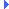FD.io VPP  v18.07.1-11-g31aa6f2 Vector Packet Processing
message_queue.c File ReferenceInclude dependency graph for message_queue.c:

Go to the source code of this file.

## Functions

svm_msg_q_tsvm_msg_q_alloc (svm_msg_q_cfg_t *cfg)
Allocate message queue. More...

void svm_msg_q_free (svm_msg_q_t *mq)
Free message queue. More...

svm_msg_q_msg_t svm_msg_q_alloc_msg (svm_msg_q_t *mq, u32 nbytes)
Allocate message buffer. More...

static svm_msg_q_ring_tsvm_msg_q_get_ring (svm_msg_q_t *mq, u32 ring_index)

static void * svm_msg_q_ring_data (svm_msg_q_ring_t *ring, u32 elt_index)

void * svm_msg_q_msg_data (svm_msg_q_t *mq, svm_msg_q_msg_t *msg)
Get data for message in queu. More...

void svm_msg_q_free_msg (svm_msg_q_t *mq, svm_msg_q_msg_t *msg)
Free message buffer. More...

static int svm_msq_q_msg_is_valid (svm_msg_q_t *mq, svm_msg_q_msg_t *msg)

int svm_msg_q_add (svm_msg_q_t *mq, svm_msg_q_msg_t msg, int nowait)
Producer enqueue one message to queue. More...

int svm_msg_q_sub (svm_msg_q_t *mq, svm_msg_q_msg_t *msg, svm_q_conditional_wait_t cond, u32 time)
Consumer dequeue one message from queue. More...

## Function Documentation

 int svm_msg_q_add ( svm_msg_q_t * mq, svm_msg_q_msg_t msg, int nowait )

Producer enqueue one message to queue.

Prior to calling this, the producer should've obtained a message buffer from one of the rings by calling svm_msg_q_alloc_msg.

Parameters
 mq message queue msg message (pointer to ring position) to be enqueued nowait flag to indicate if request is blocking or not
Returns
success status

Definition at line 143 of file message_queue.c.Here is the call graph for this function:

 svm_msg_q_t* svm_msg_q_alloc ( svm_msg_q_cfg_t * cfg )

Allocate message queue.

Allocates a message queue on the heap. Based on the configuration options, apart from the message queue this also allocates (one or multiple) shared-memory rings for the messages.

Parameters
 cfg configuration options: queue len, consumer pid, ring configs
Returns
message queue

Definition at line 20 of file message_queue.c.Here is the call graph for this function:

 svm_msg_q_msg_t svm_msg_q_alloc_msg ( svm_msg_q_t * mq, u32 nbytes )

Allocate message buffer.

Message is allocated on the first available ring capable of holding the requested number of bytes.

Parameters
 mq message queue nbytes number of bytes needed for message
Returns
message structure pointing to the ring and position allocated

Definition at line 66 of file message_queue.c.

 void svm_msg_q_free ( svm_msg_q_t * mq )

Free message queue.

Parameters
 mq message queue to be freed

Definition at line 53 of file message_queue.c.Here is the call graph for this function:

 void svm_msg_q_free_msg ( svm_msg_q_t * mq, svm_msg_q_msg_t * msg )

Free message buffer.

Marks message buffer on ring as free.

Parameters
 mq message queue msg message to be freed

Definition at line 105 of file message_queue.c.

 static svm_msg_q_ring_t* svm_msg_q_get_ring ( svm_msg_q_t * mq, u32 ring_index )
inlinestatic

Definition at line 85 of file message_queue.c.Here is the caller graph for this function:

 void* svm_msg_q_msg_data ( svm_msg_q_t * mq, svm_msg_q_msg_t * msg )

Get data for message in queu.

Parameters
 mq message queue msg message for which the data is requested
Returns
pointer to data

Definition at line 98 of file message_queue.c.Here is the call graph for this function:

 static void* svm_msg_q_ring_data ( svm_msg_q_ring_t * ring, u32 elt_index )
inlinestatic

Definition at line 91 of file message_queue.c.Here is the caller graph for this function:

 int svm_msg_q_sub ( svm_msg_q_t * mq, svm_msg_q_msg_t * msg, svm_q_conditional_wait_t cond, u32 time )

Consumer dequeue one message from queue.

This returns the message pointing to the data in the message rings. The consumer is expected to call svm_msg_q_free_msg once it finishes processing/copies the message data.

Parameters
 mq message queue msg pointer to structure where message is to be received cond flag that indicates if request should block or not
Returns
success status

Definition at line 150 of file message_queue.c.Here is the call graph for this function:

 static int svm_msq_q_msg_is_valid ( svm_msg_q_t * mq, svm_msg_q_msg_t * msg )
static

Definition at line 125 of file message_queue.c.Here is the caller graph for this function: